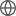Old Web
English
We present the bright (V$_{mag} = 9.12$), multi-planet system TOI-431, characterised with photometry and radial velocities. We estimate the stellar rotation period to be $30.5 \pm 0.7$ days using archival photometry and radial velocities. TOI-431b is a super-Earth with a period of 0.49 days, a radius of 1.28 $\pm$ 0.04 R$_{\oplus}$, a mass of $3.07 \pm 0.35$ M$_{\oplus}$, and a density of $8.0 \pm 1.0$ g cm$^{-3}$; TOI-431d is a sub-Neptune with a period of 12.46 days, a radius of $3.29 \pm 0.09$ R$_{\oplus}$, a mass of $9.90^{+1.53}_{-1.49}$ M$_{\oplus}$, and a density of $1.36 \pm 0.25$ g cm$^{-3}$. We find a third planet, TOI-431c, in the HARPS radial velocity data, but it is not seen to transit in the TESS light curves. It has an $M \sin i$ of $2.83^{+0.41}_{-0.34}$ M$_{\oplus}$, and a period of 4.85 days. TOI-431d likely has an extended atmosphere and is one of the most well-suited TESS discoveries for atmospheric characterisation, while the super-Earth TOI-431b may be a stripped core. These planets straddle the radius gap, presenting an interesting case-study for atmospheric evolution, and TOI-431b is a prime TESS discovery for the study of rocky planet phase curves.